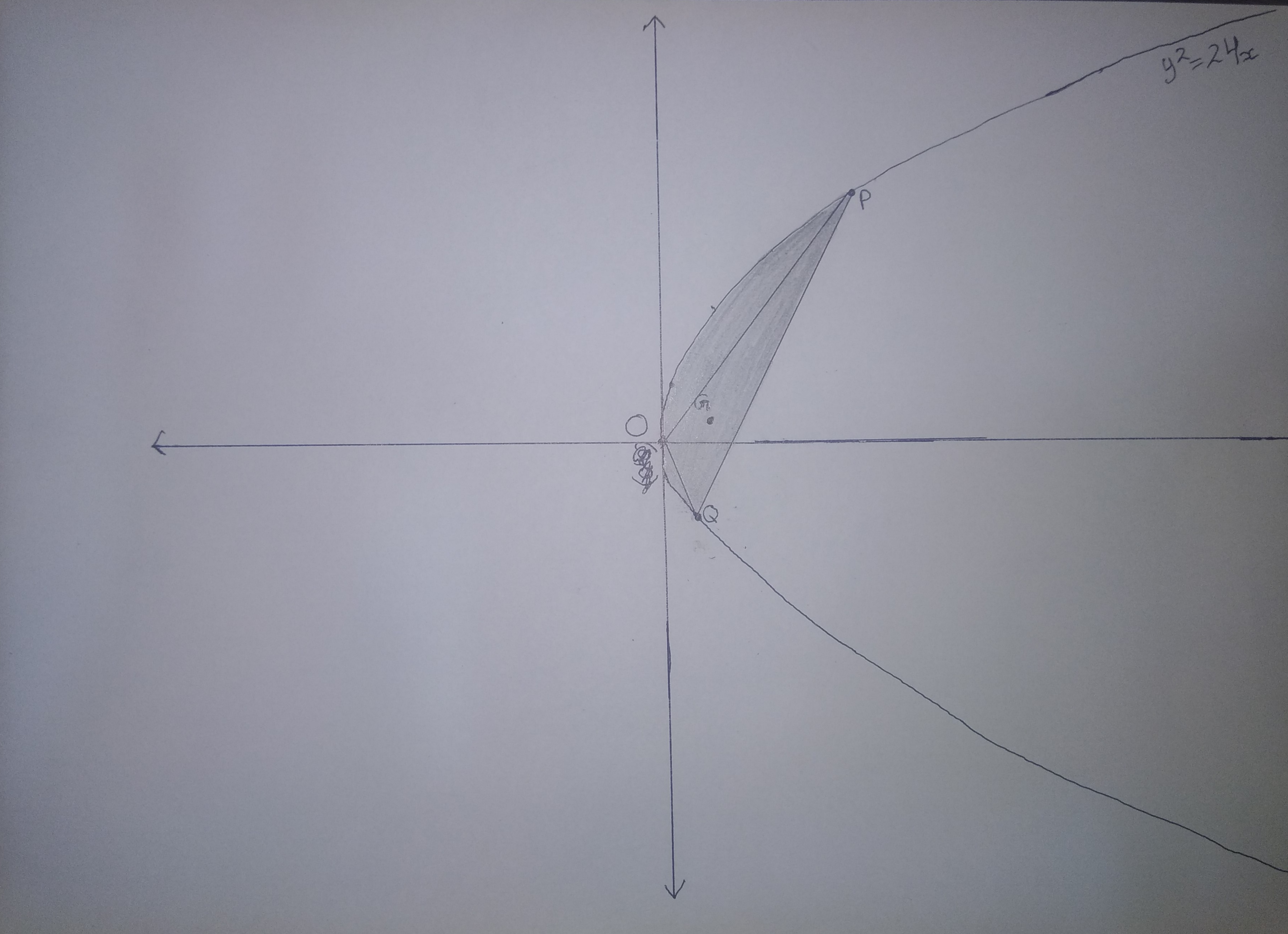# Areal Parabolic CentroidFor a Parabola $y^2 = 24x$, consider all chords $PQ$ of the parabola such that the chords make a constant area $A \gt 12$ with the parabola. The vertex of above parabola is $O$.

Find locus of centroid of $\Delta OPQ$. Length of Latus Rectum of locus is $L$ and coordinates of focus of locus is $S(x, y)$.

If $L = a, x \gt b, y = c$, Enter answer as $a + b + c$

Try similar problem

All of my problems are original

×